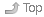# Week 04 Notes for CST8281 - Fall 2011

Fall 2011 - September to December 2011 - Updated 2020-11-12 14:48 EST

# 1 Midterm Test #1 - 15%Index• Fill out the Doodle to pick the best date.
• For full mark credit, read the Test Instructions for important directions on how to enter your answers on the mark-sense forms.
• Calculators are not permitted during the first midterm test. You will benefit from knowing the powers of two from 2^(-4) to 2^16 and the decimal and binary values of the hexadecimal digits from zero to fifteen. Of course you can work them out; however, having at least some of them memorized will make things go faster for you on the test. (Remember that hexadecimal “A” = decimal 10 = binary 1010.)

# 2 Lecture Notes for This WeekIndex## 2.2 From Blackboard Course DocumentsIndexThese documents have restricted distribution and cannot be put on the Course Home Page.

• 01.ppt - Introduction (vonNeumann, prefixes, reciprocal)
• 02.ppt - Data Representation
• omit “Booth’s algorithm” slides 50-52
• ignore slides 63-70 (the “simplified” model)
• slide 72 is wrong: IEEE 754 +0.0 is equal to -0.0
• ignore slides 75-76 (floating-point multiplication)
• use my web pages and assignments instead for floating-point info
• you do not need to know how to do math with sign-magnitude numbers
• you do need to know how to convert them to/from decimal
• you do not need to know how to do math with one’s complement numbers
• you do need to know how to convert them to/from decimal
• you need to know how to do addition with two’s complement numbers
• you also need to know how to convert them to/from decimal
• you do not have to multiply or divide or subtract any binary numbers
• omit most of the math in 2.8 “Error Detection and Correction”
• omit slides 94-100
• omit slides 103-115
• 05.ppt - instruction set architectures
• only read endian-ness on slide 7
• omit the rest of this slide set

## 2.4 From the Classroom Whiteboard/ChalkboardIndex• Your in-class notes go here.

• Midterm Test One Date (Doodle Poll): check your EMail or look for the Announcement on Blackboard.

• Date extension granted for Assignment #03.

• Write 4-bit 1010(2) as hex, unsigned, s/m, one’s, two’s, and bias-8

• Answer: A 10 -2 -5 -6 +2
• now try 1100(2)
• 11 bits holds 2^11 numbers (2^11 = 2^1 * 2^10 = 2 * 1024 = 2048 numbers)

• if all positive (unsigned): 0 to 2047
• if two’s complement, half are negative, so -1024…-1,0,+1…+1023
• Integers have no gaps. If range is -1024 to +1023, no integers are missing.

• Multi-byte integers and endian-ness 080_byte_order_endian.html and 05.ppt #7

### 2.4.1 Floating PointIndex• There are an infinite number of floating point numbers between any two numbers!
• We normalize floating point numbers to ensure a unique representation
• Floating point is expressed in three pieces and stored in three pieces:
• e.g. 3.1415(10) * 10^0 or 11.101(2) * 2^10
• sign (positive or negative)
• mantissa or significand
• exponent
• Only the sign can be represented accurately. In a computer, we have a limit on the number of bits in the significand, and in the exponent.
• floating point numbers have two types of errors
• missing bits in the significand will mean loss of precision
• missing bits in the exponent will mean loss of range
• IEEE 754 Single Precision: one bit sign, eight bit exponent, 23 bit significand
``````Author:
| Ian! D. Allen  -  idallen@idallen.ca  -  Ottawa, Ontario, Canada